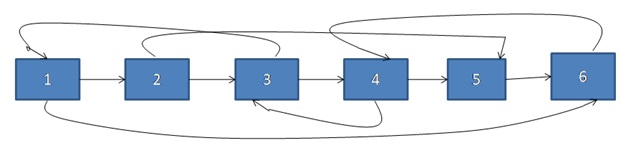# Clone a linked list with next and random pointer using C++ program

In this article, we are going to learn how to clone a linked list with next random pointer? This article contains problem statement, explanation, algorithm, C++ implementation and output.
Submitted by Souvik Saha, on May 06, 2019

Problem statement:

Given a linked list, there are two node pointers one point to the next node of the linked list and another is the random pointer which points to any random node of that linked list. Your task is to make a clone a linked list of that linked list.

Example:

Input:Output

Make a same copy of that linked list.

Algorithm:

Node structure for random pointer:

```    Node temp
{
Int data;
Node* next;
Node* random=NULL;
}
```

To solve that problem we follow this algorithm:

1. First, we copy the next pointers and make a linked list.
2. In the second iteration, we copy the random pointers of that linked list.

Using this algorithm the time complexity is O(n).

C++ implementation:

```#include <bits/stdc++.h>
using namespace std;

struct node {
int data;
node* next;
node* random;
};

//Create a new node
struct node* create_node(int x)
{
struct node* temp = new node;
temp->data = x;
temp->next = NULL;
temp->random = NULL;
return temp;
}

void push_random(node* head, int x, int y)
{
while (temp1) {
if (temp1->data == x) {
break;
}
temp1 = temp1->next;
}
while (temp2) {
if (temp2->data == y) {
break;
}
temp2 = temp2->next;
}
temp1->random = temp2;
}

//Enter the node into the linked list
{
struct node* store = create_node(x);
return;
}
while (temp->next) {
temp = temp->next;
}
temp->next = store;
}

{
struct node* store = create_node(0);
struct node* curr = store;
while (temp) {
curr->next = create_node(temp->data);
temp = temp->next;
curr = curr->next;
}
curr = store;
while (temp) {
curr->random = temp->random;
curr = curr->next;
temp = temp->next;
}
store = store->next;
return store;
}

//Print the list
{
while (temp) {
cout << temp->data << " ";
temp = temp->next;
}
}

int main()
{
struct node* l = NULL;
push(&l, 1);
push(&l, 2);
push(&l, 3);
push(&l, 4);
push(&l, 5);
push(&l, 6);
srand(time(0));
for (int i = 1; i <= 6; i++) {
int r = (rand() % 6) + 1;
push_random(l, i, r);
}
cout << "Original list" << endl;
print(l);
struct node* c = copy(l);
cout << "\nCopy list" << endl;
print(c);

return 0;
}
```

Output

```Original list
1 2 3 4 5 6
Copy list
1 2 3 4 5 6
```